# Substitution method

Solve goniometric equation:

sin4 θ - 1/cos2 θ=cos2 θ - 2

Result

θ = (Correct answer is: k*pi)#### Solution: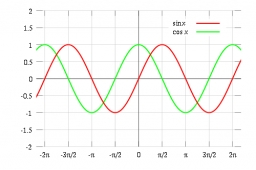We would be pleased if you find an error in the word problem, spelling mistakes, or inaccuracies and send it to us. Thank you!Tips to related online calculators
Looking for help with calculating roots of a quadratic equation?
Do you have a linear equation or system of equations and looking for its solution? Or do you have quadratic equation?
Most natural application of trigonometry and trigonometric functions is a calculation of the triangles. Common and less common calculations of different types of triangles offers our triangle calculator. Word trigonometry comes from Greek and literally means triangle calculation.

## Next similar math problems:

• TrigonometryIf you know that cos(γ) = sin (806°), what is the angle γ?
• Completing squareSolve the quadratic equation: m2=4m+20 using completing the square method
• Q-Exam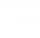If tg α = 9.6, Calculating sin α, cos α, cotg α .
• CotangentIf the angle α is acute, and cotg α = 1/3. Determine the value of sin α, cos α, tg α.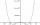By introducing a new variable solve biquadratic equation: ?
• CosineThe point (3, 4) is on the terminal side of angle θ. cos θ = ?
• Solve equationsolve equation: ?
• Right triangle eq2Find the lengths of the sides and the angles in the right triangle. Given area S = 210 and perimeter o = 70.
• Non linear eqsSolve the system of non-linear equations: 3x2-3x-y=-2 -6x2-x-y=-7
• Find the 2Find the term independent of x in the expansion of (4x3+1/2x)8
• Unknown number 7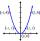Calculate unknown number whose 12th power when divided by the 9th power get a number 27 times greater than the unknown number. Determine the unknown number.
• Triangle in a squareIn a square ABCD with side a = 6 cm, point E is the center of side AB and point F is the center of side BC. Calculate the size of all angles of the triangle DEF and the lengths of its sides.
• EQ2Solve quadratic equation: ?
• Height of the arc - formula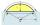Calculate the height of the arc if the length of the arc is 77 and chord length 40. Does exist a formula to solve this?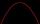Solve pure quadratic equation ?.The sum of three numbers in GP (geometric progression) is 21 and the sum of their squares is 189. Find the numbers.Area of the triangle is 12 cm square. Angle ACB = 30º , AC = (x + 2) cm, BC = x cm. Calculate the value of x.